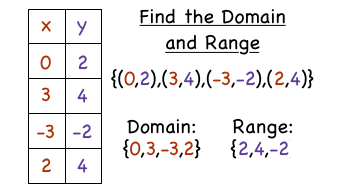# What is Domain and Range of a Function?

Apr 7, 2018

First, let's define a function:
A function is a relationship between the $x$ and $y$ values, where each $x$-value or input has only one $y$-value or output .

Domain: all x-values or inputs that have an output of real $y$-values.

Range: the y-values or outputs of a functionFor example,